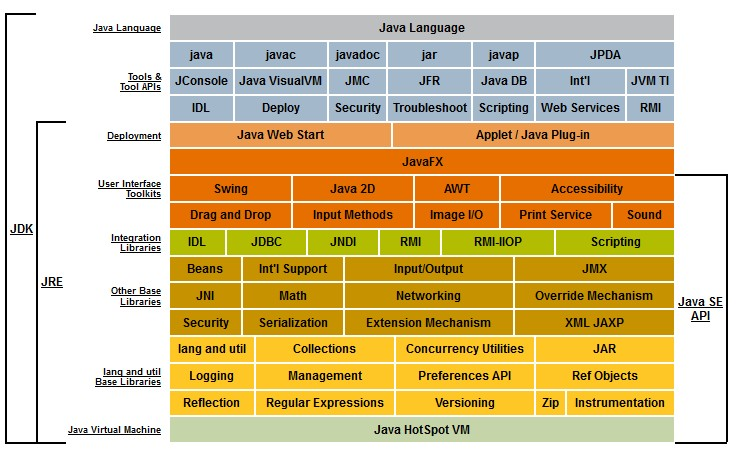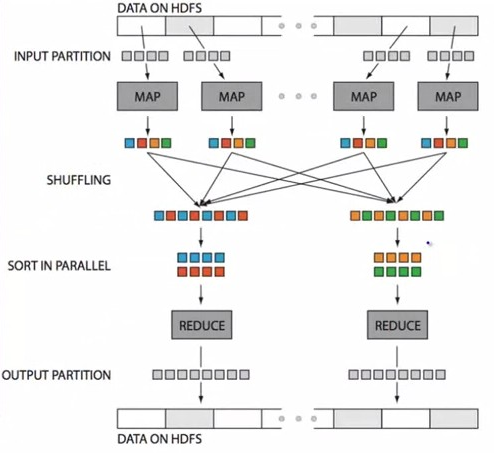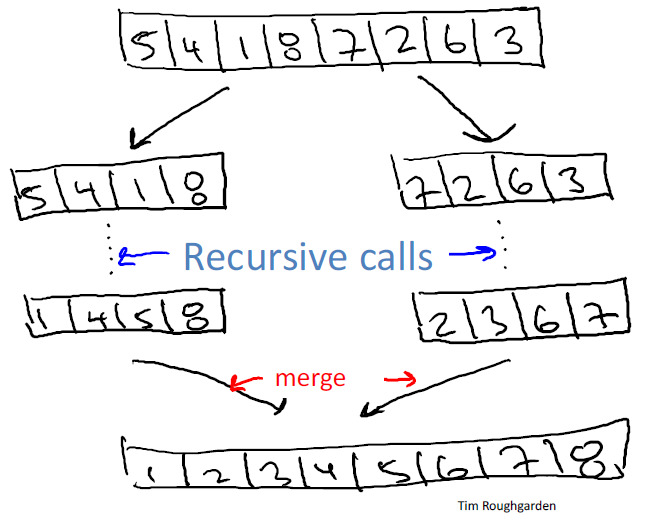# Ordinal Data - Sorting (Problem / Algorithm)

• Input: array of n numbers, unsorted.
• Output: array of n numbers, sorted (from smallest to largest)

Possible Assumption:

• numbers are distinct
• with duplicates, the problem can even be easier

Sorting has complexity O(n log n) but repeated sorting can be avoided with a better data structure

## Illustration

Manual sorting and counting of 4 million London Underground tickets in 1939 to analyse train use across the network.

## Algorithm

Recommended Pages(Data Processing|Data Integration)

Data processing is a more general term for manipulating data whereas data integration is the integration of data between two systems. Data Integration has roughly two data processing model: Model...Data Processing - Operations / Operator

A data processing function takes an input and creates an output in a pipeline. transition in Automata functional interface in Functional Programming Filter in Data Processing (Shell and Log Pipeline)...Data Warehousing - 34 Kimball Subsytems

This page takes back the Kimball Datawarehouse 34 Subsystem as a table of content and links them to a page on this website....Function - Order

Ordering is the process of finding the position of an element of ordinal data type in a sequence. Order and Equivalence are a special kind of binary relation (ie relation between two entities) With the...Java - Compare Object (Comparable) (Collection Sort)

Sort problem in java In order to sort a collection, you need to compare each element of the collection. To do this, you can implement the java/lang/ComparableComparable interface and use a...Language - Equality

The Equality operators model the Equivalence relation: equality is parallel to (for affine spaces) is in bijection with isomorphy The Equality operators are a subset of the comparison operator....Map Reduce - Sort

sort in a mapreduce is a operation that happens after a shuffling.Ordinal Data - Comparison Operator (Order, Equality)

A comparison operator is a class of operators that model: a order or a equivalence relationship A comparison operator test the order relationship between two operands and returns a Boolean. The...Ordinal Data - Heapsort sorting algorithm

The heap sorting algorithm is a sorting algorithm based on a binary heap (ie binary tree) introduced by J. W. J. Williams in 1964 HeapsortOrdinal Data - Merge sort Algorithm

John von Neumann invented merge sort in 1945. It requires O(n log n) comparisons. A sorting algorithm for rearranging lists (or any other data structure that can only be accessed sequentially, e.g. file...